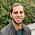Skip to main content

### Happy π day! Estimating Pi by graphing random numbers with Stata.

√-1 2^3 Σπ and it was delicious (because it was of the pizza variety).

My obligatory March 14 Pi  post involves estimating Pi by finding the proportion of randomly plotted points inside a square that are also inside a circumscribed circle. The larger the number of points we plot using this method, the closer to Pi we get (sort of Pi estimation by  simulation in Stata (I know, I know, it should have been programmed in PYthon)).

You can approximate a Pi calculation via boring methods like:
• pressing the π on your TI-83 calculator ,
• calculating fractions like 22/7 or 355/113 ,
• calculating the log(6)^log(5)^log(4)^log(3)^log(2),  or whatnot.
e.g.,

. di  22/7
3.1428571

. di  355/113
3.1415929

. di  log(6)^(log(5)^(log(4)^(log(3)^log(2))))
3.1415774

or just cheat and plot  circles via -tw- functions or -graph pie-, etc in Stata.

However  (assuming you aren't Yasumasa Kanada and Daisuke Takahashi from the U of Tokyo with server cycles to spare)  another approach for calculating Pi is to _draw a square circumscribing a circle and then randomly plot points inside the square and calculate the proportion of those random points that fell into the circle portion_.  This proportion is roughly Pi (especially if you plot a lot of random points).  You can try this at home:  Get a crayola and create the figure on the right and plot lots of points-- just be sure that your hand is a random number generator for plotting the points within the square -- and then calculate:

((number of points inside unit circle)/(total # pts)) x Area (or 4)

The program code included at the bottom of this post does this in Stata for you.  It first plots a circle circumscribed by a square (you can access just this graph (shown on the right) by running the option 'basegraph') and then it plots the # of random points you specify and calculates the proportion insides the circle; this approximates Pi ( increasingly as the # of random points -->  ∞ ).  Algorithms like these, which use random numbers to approximate deterministic outcomes, are often called Monte Carlo methods/simulations.

Example: Plotting 105k points gets us within about .0061 of Pi (in the first 16 digits), the output is:

AFTER GENERATING  105500   RANDOM POINTS:
SIMULATED pi is: 3.14775355450237
REAL pi is :     3.141592653589793
Diff is :        -.0061609009125769

If you run the code below you can watch the simulated random points that are plotted increase and you'll notice the improvement in the estimated Pi value in the graph caption as well as the output in the results window.

I thought it would be irrational to exclude a GIF of the example code running on my computer:

Here's the program to simulate Pi via graphing methods in Stata:

### Comments

1.Glad to share my R version! Hope you find it useful ;)

set.seed(0)
options(digits=20)
iters <- 1e8
r <- 1

points <- data.frame(
x = runif(iters, -r, r),
y = runif(iters, -r, r))
points\$d <- sqrt(points\$x^2 + points\$y^2)
points\$type <- ifelse(points\$d < r, "c", "s")

picalc <- 4 * length(points\$type[points\$type=="c"]) / iters
abs(pi - picalc)

It returns a 1.87e-05 error in around 30 seconds :D